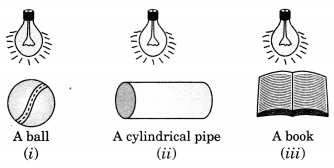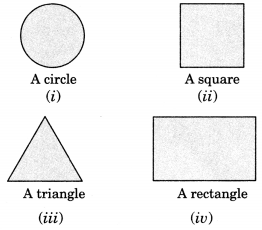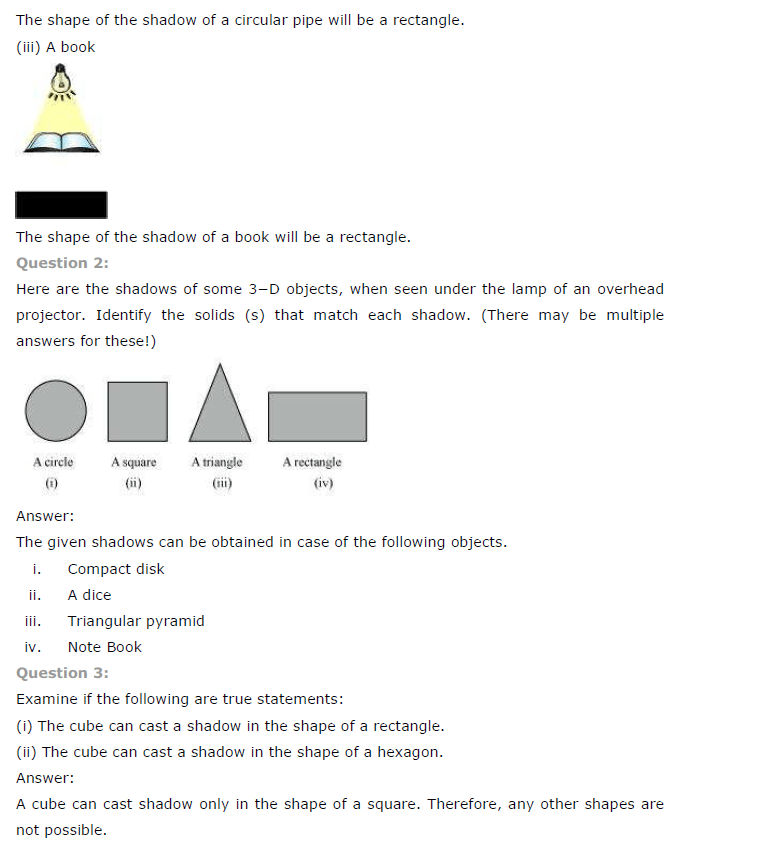# NCERT Solutions for Class 7 Maths Chapter 15 Visualising Solid Shapes Ex 15.4

NCERT Solutions for Class 7 Maths Chapter 15 Visualising Solid Shapes Exercise 15.4
Ex 15.4 Class 7 Maths Question 1.
A bulb is kept burning just right above the following solids. Name the shape of the shadows obtained in each case. Attempt to give a rough sketch of the shadow. (You may try to experiment first and then answer these questions).Solution:
When the light falls just above the solids.
(i) A ball; the shadow looks like a circle.
(ii) A cylindrical pipe; the shadow looks like nearly rectangular.
(iii) A book; the shadow looks like nearly rectangular.

Ex 15.4 Class 7 Maths Question 2.
Here are the shadows of some 3-D objects, when seen under the lamp of an overhead projector. Identify the solid(s) that match each shadow. (There may be multiple answers for these!)Solution:
(i) The given shadow corresponds to a sphere.
(ii) The given shadow corresponds to a cube.
(iii) The given shadow corresponds to a pyramid.
(iv) The given shadow corresponds to a cuboid or a cylinder.

Ex 15.4 Class 7 Maths Question 3.
Examine if the following are true statements:
(i) The cube can cast a shadow in the shape of a rectangle.
(ii) The cube can cast a shadow in the shape of a hexagon.
Solution:
(i) The given statement is true.
(ii) The given statement is false.+